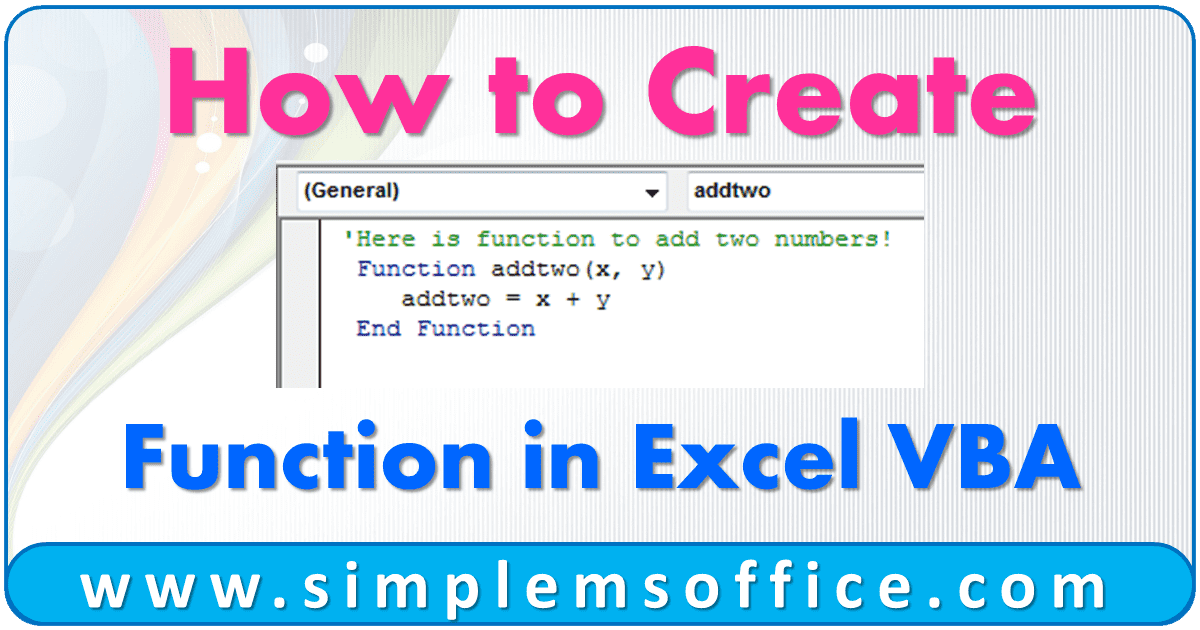# How to Create Function in Excel VBAIf you have used excel, you also probably used some excel formulas like sum, average, count, etc.

But do you know that these formulas are pre-loaded functions in excel?

Yes, these all are functions and you can also create your own functions in excel using VBA coding.

Creating a function for you will be very easy because we will guide you step by step. After completing this post, you will be able to create your own functions according to your need.

Let’s start….

### We will create a function to add two numbers

Step 1: Open New Excel File and Press the key Alt+F11. It will open Microsoft Visual Basic for Applications Window.

Step 2: Go to Insert >> Module menu. You will see a new window in which you have to write the function.

Step 3: Copy the below code and paste it into the code window:

 1234 'Here is function to add two numbers!  Function addtwo(x, y)     addtwo = x + y  End Function

Step 4: Go to the File menu and Click on the last option Close and Return to Microsoft Excel or press Ctrl+Q.

That’s all. Your function is ready. Now question is, how to use this function? So go to the next step.

Step 5: Now in the Excel sheet, write a function like a formula in cell A1.

Step 6: Press Enter, and you will see the result as 8.

Congratulations!!!

You have created an add-in function. So, below are some points you have to look up.

1. You have to tell the function, what you want to input. Like “(x,y)” following the function name.
1. You tell the function with a line that (“addtwo”) is equal to the calculation (“x+y”).
1. VBA automatically understands the script coding, comment, and function. Like after adding the script line, when you will press enter it will automatically color-code the text and complete the syntax.

For example, the line “End Function” will automatically insert when you write Function and function name in the first line. Because VBA understands its script and syntax structure.

Step 7: After adding the code, save the file. Go to the File menu and click on Save or press Ctrl+S.

Note:- If you want to edit VBA code, then follow Step 1 and after editing, follow Step 7.